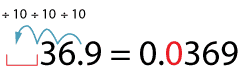#### Dividing decimal numbers by 10, 100 or 1000

Dividing decimal numbers by 10, 100 or 1000 follows the same procedure used for dividing whole numbers by 10, 100 or 1000.

1.4 ÷ 10: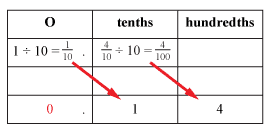Detailed description

14 ÷ 10 = 1.4

1.4 ÷ 100: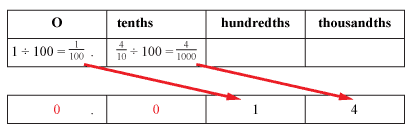Detailed description

We place zeroes in the spaces to say that there are 'no tenths' and 'no ones'.
1.4 ÷ 100 = 0.014

You might have noticed another shortcut:

\begin{align*} 36.9 \div 10 &= (30 \div 10) + (6 \div 10) + (\frac{9}{10} \div 10) \\ &= 3 + \frac{6}{10} + \frac{9}{100} \\ &= 3.69 \end{align*}

When you divide a decimal by ten, the decimal point moves one place to the left.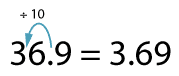When you divide a decimal by 100, the decimal point moves two places to the left.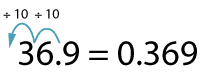When you divide a decimal by 1000, the decimal point moves three places to the left and you fill the new tenths place with a zero to say that there are 'no tenths'.